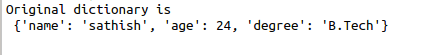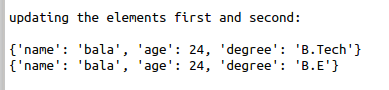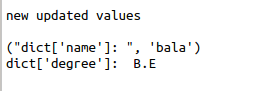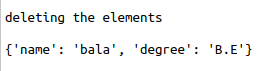• #5, First Floor, 4th Street Dr. Subbarayan Nagar Kodambakkam, Chennai-600 024 Landmark : Samiyar Madam
• pro@slogix.in
• +91- 81240 01111

### How to implement Hash Tables using python dictionaries?

###### Description

To implement the hash tables using python3.

###### Hash Table:

Hash tables are a type of data structure in which the address or the index value of the data

element is generated from a hash function.

That makes accessing the data faster as the index value behaves as a key for the data value.

The keys of the dictionary are hashable

###### Sample Code

#sample dictionary
dict={‘name’:’sathish’,’age’:24,’degree’:’B.Tech’}
print(“Original dictionary is\n”,dict)
print(“\n”)

#accessing values from dictionary
print(“Accessing the values\n”)
print (“dict[‘name’]: “, dict[‘name’])
print (“dict[‘age’]: “, dict[‘age’])
print(“\n”)

#update existing entry
print(“updating the elements first and second:\n”)
dict[‘name’] = ‘bala’;
print(dict)

dict[‘degree’] = ‘B.E’;
print(dict)
print(“\n”)

#printing the new dict
print(“new updated values\n”)
print((“dict[‘name’]: “, dict[‘name’]))
print(“dict[‘degree’]: “, dict[‘degree’])
print(“\n”)

#delete dictionary elements
print(“deleting the elements\n”)
del dict[‘age’];
print(dict)

###### Screenshots